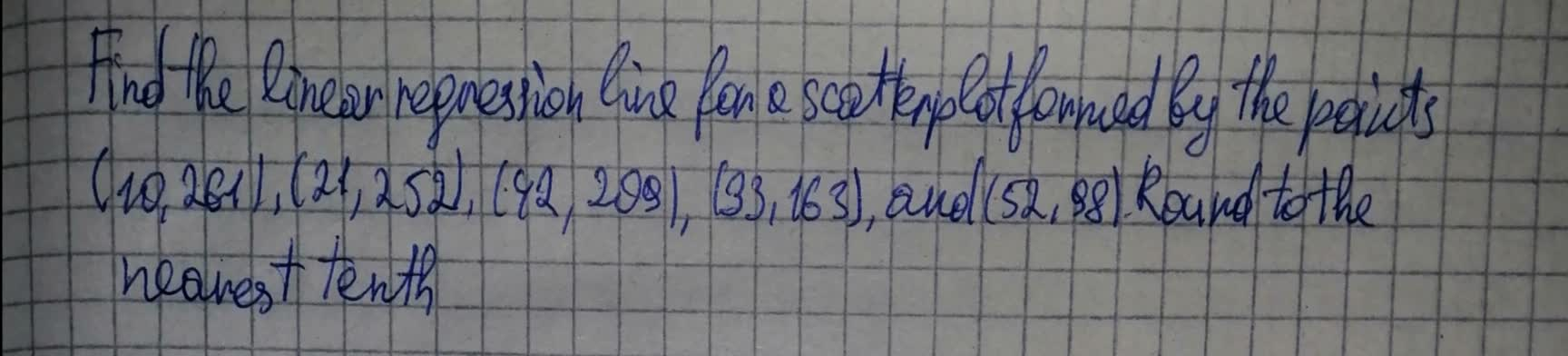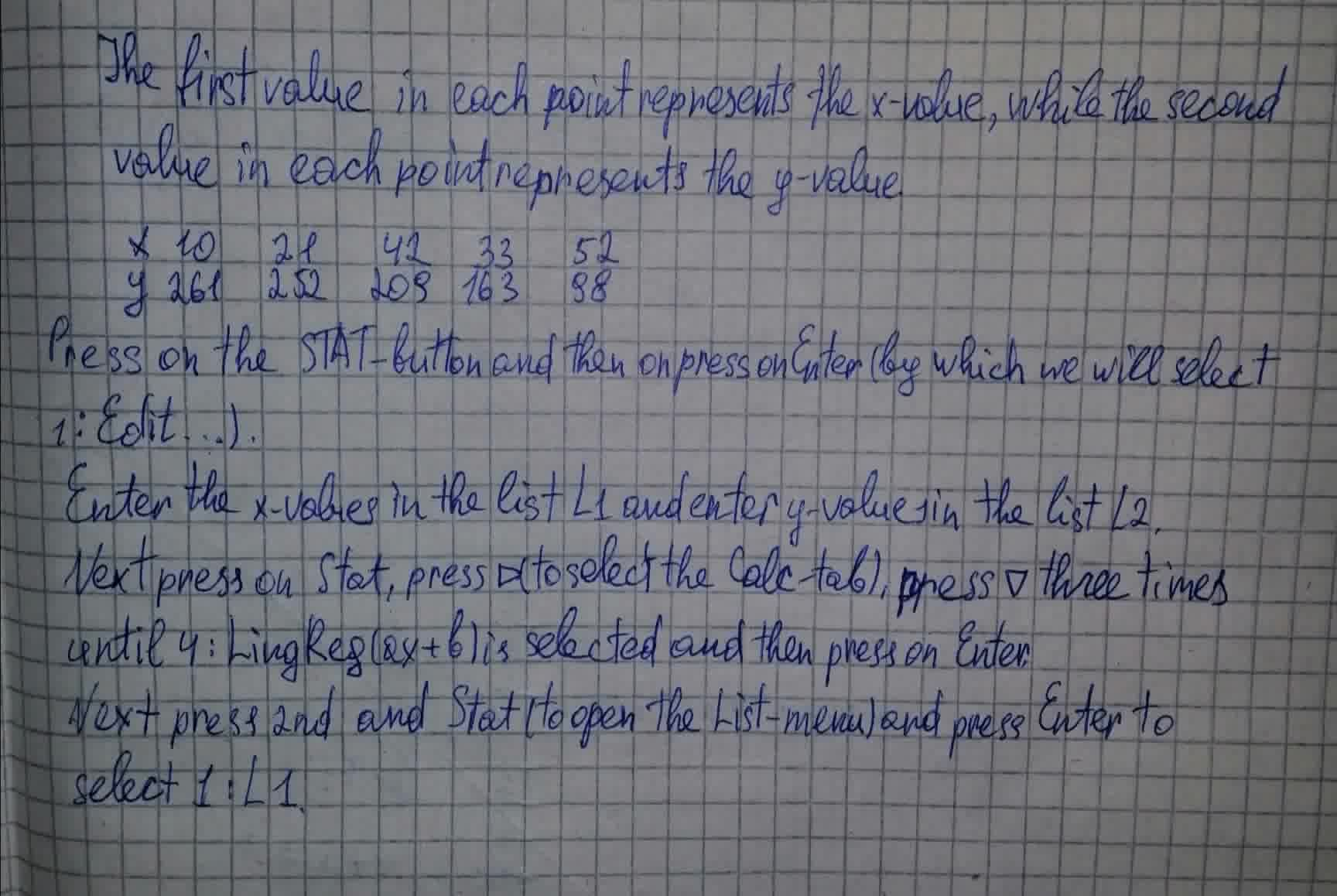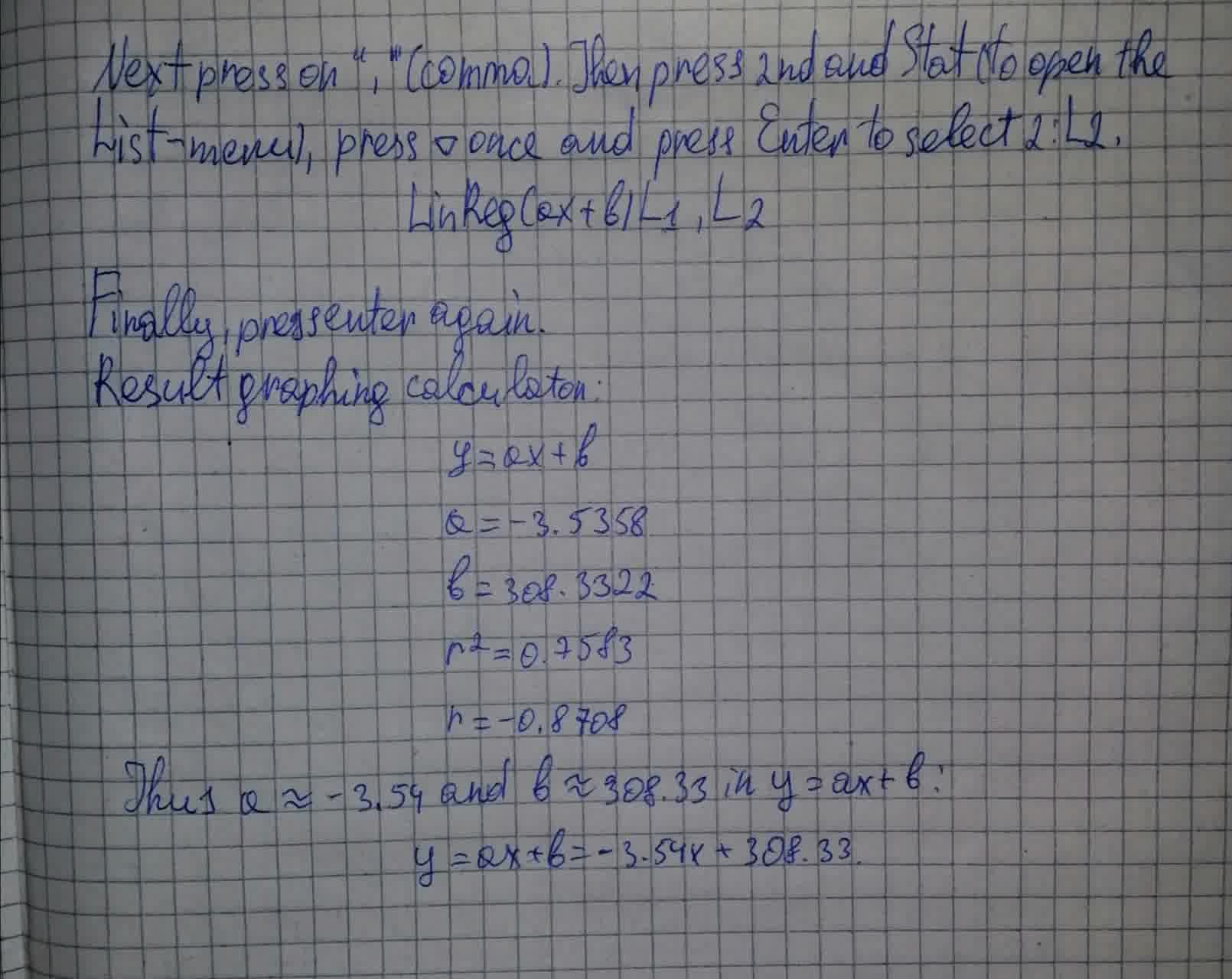# Find the linear regression line for a scatterplot formed by the points (10, 261), (21, 252), (42, 209), (33, 163), and (52, 98).Line 2021-07-23 Answered
Find the linear regression line for a scatterplot formed by the points (10, 261), (21, 252), (42, 209), (33, 163), and (52, 98). Round to the nearest tenth.You can still ask an expert for help

• Questions are typically answered in as fast as 30 minutes

Solve your problem for the price of one coffee

• Math expert for every subject
• Pay only if we can solve itSchepperJ

Solution to problem: